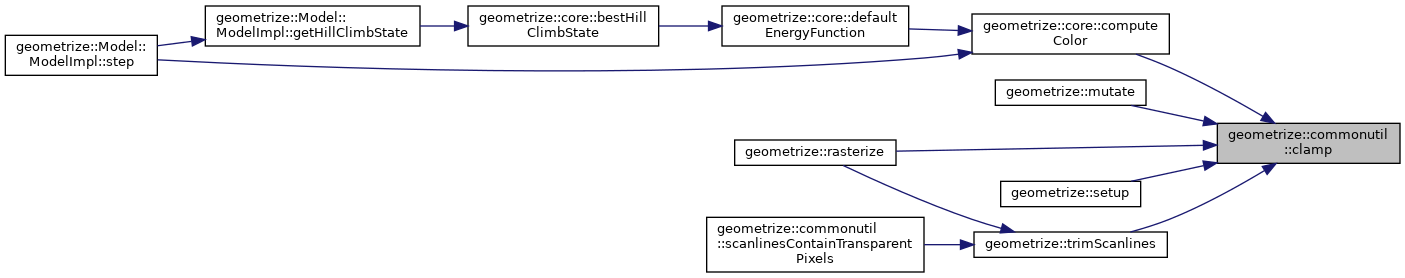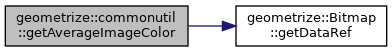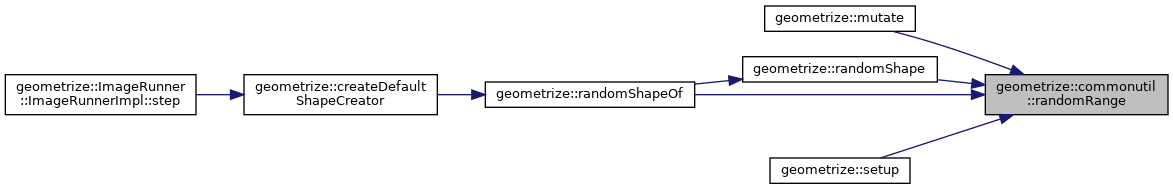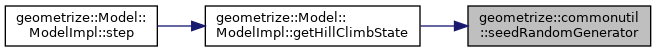Geometrize  1.0 C++ library for geometrizing images into geometric primitives
geometrize::commonutil Namespace Reference

## Functions

void seedRandomGenerator (std::uint32_t seed)
seedRandomGenerator Seeds the (thread-local) random number generators. More...

std::int32_t randomRange (std::int32_t min, std::int32_t max)
randomRange Returns a random integer in the range, inclusive. Uses thread-local random number generators under the hood. To ensure deterministic shape generation that can be repeated for different seeds, this should be used for shape mutation, but nothing else. More...

geometrize::rgba getAverageImageColor (const geometrize::Bitmap &image)
getAverageImageColor Computes the average RGB color of the pixels in the bitmap. More...

template<typename T >
clamp (const T &value, const T &lower, const T &upper)
clamp Clamps a value within a range. More...

## Variables

std::uniform_int_distribution
< std::int32_t >
pick

## Function Documentation

template<typename T >
 T geometrize::commonutil::clamp ( const T & value, const T & lower, const T & upper )

clamp Clamps a value within a range.

Parameters
 value The value to clamp. lower The lower bound of the range. upper The upper bound of the range.
Returns
The clamped value.
42 {
43  return (std::max)(lower, (std::min)(value, upper));
44 }

Here is the caller graph for this function:geometrize::rgba geometrize::commonutil::getAverageImageColor ( const geometrize::Bitmap & image )

getAverageImageColor Computes the average RGB color of the pixels in the bitmap.

Parameters
 image The image whose average color will be calculated.
Returns
The average RGB color of the image, RGBA8888 format. Alpha is set to opaque (255).
31 {
32  const std::vector<std::uint8_t>& data{image.getDataRef()};
33  const std::size_t size{data.size()};
34  if(size == 0) {
35  return geometrize::rgba{0, 0, 0, 0};
36  }
37
38  const std::size_t numPixels{size / 4U};
39
40  std::uint32_t totalRed{0};
41  std::uint32_t totalGreen{0};
42  std::uint32_t totalBlue{0};
43  for(std::size_t i = 0; i < size; i += 4U) {
44  totalRed += data[i];
45  totalGreen += data[i + 1U];
46  totalBlue += data[i + 2U];
47  }
48
49  return geometrize::rgba{
50  static_cast<std::uint8_t>(totalRed / numPixels),
51  static_cast<std::uint8_t>(totalGreen / numPixels),
52  static_cast<std::uint8_t>(totalBlue / numPixels),
53  static_cast<std::uint8_t>(UINT8_MAX)
54  };
55 }
const std::vector< std::uint8_t > & getDataRef() const
getDataRef Gets a reference to the raw bitmap data.
Definition: bitmap.cpp:35
The rgba struct is a helper for manipulating RGBA8888 color data.
Definition: rgba.h:12

Here is the call graph for this function:static thread_local std::mt19937 geometrize::commonutil::mt ( std::random_device{} () )
static
 std::int32_t geometrize::commonutil::randomRange ( std::int32_t min, std::int32_t max )

randomRange Returns a random integer in the range, inclusive. Uses thread-local random number generators under the hood. To ensure deterministic shape generation that can be repeated for different seeds, this should be used for shape mutation, but nothing else.

Parameters
 min The lower bound. max The upper bound.
Returns
The random integer in the range.
25 {
26  assert(min <= max);
27  return pick(mt, std::uniform_int_distribution<std::int32_t>::param_type{min, max});
28 }
static thread_local std::uniform_int_distribution< std::int32_t > pick
Definition: commonutil.cpp:17

Here is the caller graph for this function:void geometrize::commonutil::seedRandomGenerator ( std::uint32_t seed )

seedRandomGenerator Seeds the (thread-local) random number generators.

Parameters
 seed The random seed.
20 {
21  mt.seed(seed);
22 }